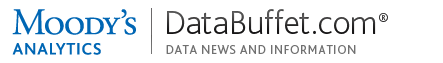Logout / Access Other productsGet live help Monday-Friday from 7:00AM-6:00PM ET (11:00AM-10:00PM GMT)  •  Contact Us
Check out our new FAQ section!
AuthorPhillip Thorne
Question

How can I create custom formulas in a Data Buffet basket to perform arbitrary computation on native series?

A Data Buffet basket can contain four types of expressions:

• Single mnemonic (one series)
• Single concept using geo list (may expand to multiple series)
• Wild card (may expand to multiple series)
• Formula (one synthetic series)

A formula mathematically combines one or more native time series to emit a single synthetic time series.  Some of the operations can be performed identically post-download, but to do so within Data Buffet can simplify your workflow.  Formulas also permit time series manipulation for which external spreadsheets are ill-suited:

• Convert operands to a single compatible frequency
• Index (extract) single periods
• Use period lags and leads

To differentiate a formula from the other three types of expression, it must be enclosed in parentheses:

• Single mnemonic: ET.PA
• Formula: (ET.PA + ET.DE)
• Wrong (lacks parentheses): ET.PA + ET.DE

Formulas can be used in View, Basket, Chart and Map modes.

Caveats

Formulas cannot embed wild cards or geo lists:

• Wrong: (ET.new_england.lst + ET.mid_atlantic.lst)
• Wrong: (FET.M^^^ / 12)

Formulas cannot be used as geo lists:

• Geo list: (ME+NH+VT+MA+CT+RI)
• Formula using illegal geo list: (RET2371Q.my_agg.lst + RET2373Q.my_agg.lst)

Data Buffet formulas do not behave like the "aggregate functions" of SQL.  You cannot "specify a bunch of series" and then operate on them as a group.  Instead, if you need to "cross-aggregate" "set of concepts" with "set of geographies," you can download the series to Microsoft Excel with Moody's Analytics Power Tools, and then write custom worksheet formulas around them.

Operators

• Addition, subtraction, multiplication and division (+ - * /).
• The exponentiation operator is (**)
• Date indexing (extracting a single period from a time series) uses square brackets, e.g. ET.US[Jan1990]
• Control order of execution with parentheses.
• Formulas are case-insensitive.
• Formulas ignore whitespace.

Dates can be expressed in these formats:

• Daily and weekly = 25dec2010
• Monthly = Jan1990 or 1990m1
• Quarterly = 1990q2
• Annual = 2010

Debugging

Formulas most often fail because:

• The enclosing parentheses were omitted.
• The mnemonics are mis-spelled, so the series does not exist.
• The series has been renamed, so the mnemonic is no longer applicable.
• Values do not exist (i.e., are ND) for part of the date range.
• You combined series with different frequencies without a CONVERT function.

Do not try to write a complicated formula all at once; instead, "grow" it. Start with a basket that downloads each component series (operand) separately; so you can verify your access and the date range. Then build each sub-expression separately.

Examples

Aggregate (sum) employment for three areas:

(ET.DE + ET.NJ + ET.PA)

Compute state-level productivity by worker (i.e., real output divided by payroll employment):

(RGDP\$Q.AL / RETQ.AL)

Index employment to a base period, to wit, January 1990:

(100 * ET.US / ET.US[Jan1990])

CAGRs of a 10-year T-note (monthly series) over 3 years (36 months):

((IRGT10YM.US[T] / IRGT10YM.US[T-36])**(1/3) - 1)